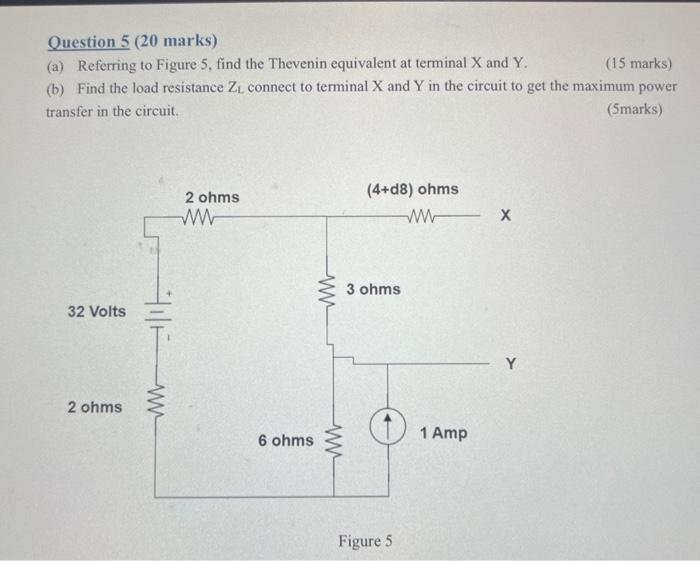# Question Question 5 (20 marks) (a) Referring to Figure 5 , find the Thevenin equivalent at terminal $$\mathrm{X}$$ and $$\mathrm{Y}$$. (15 marks) (b) Find the load resistance $$\mathrm{Z}_{\mathrm{L}}$$ connect to terminal $$\mathrm{X}$$ and $$\mathrm{Y}$$ in the circuit to get the maximum power transfer in the circuit. (5marks) Figure 5Transcribed Image Text: Question 5 (20 marks) (a) Referring to Figure 5 , find the Thevenin equivalent at terminal $$\mathrm{X}$$ and $$\mathrm{Y}$$. (15 marks) (b) Find the load resistance $$\mathrm{Z}_{\mathrm{L}}$$ connect to terminal $$\mathrm{X}$$ and $$\mathrm{Y}$$ in the circuit to get the maximum power transfer in the circuit. (5marks) Figure 5
Transcribed Image Text: Question 5 (20 marks) (a) Referring to Figure 5 , find the Thevenin equivalent at terminal $$\mathrm{X}$$ and $$\mathrm{Y}$$. (15 marks) (b) Find the load resistance $$\mathrm{Z}_{\mathrm{L}}$$ connect to terminal $$\mathrm{X}$$ and $$\mathrm{Y}$$ in the circuit to get the maximum power transfer in the circuit. (5marks) Figure 5&#12304;General guidance&#12305;The answer provided below has been developed in a clear step by step manner.Step1/2Explanation:THEVENIN Equivalent is that it can simplify any linear circuit,it can seem complex it is equal to a circuit with voltage source and series of resistors\begin{align*} \mathrm{{V}{a}{b}={V}{x}{y}} &= \mathrm{{V}{t}{h}} \end{align*}Vab=I2(R)32=I(2+2)I=8 ampsVxy=8^2( ... See the full answer